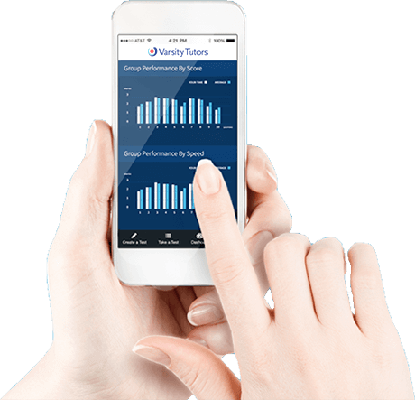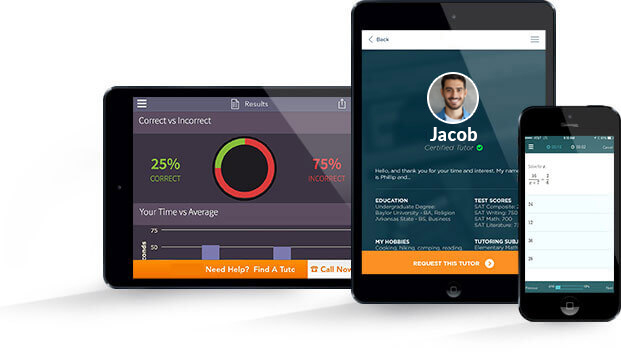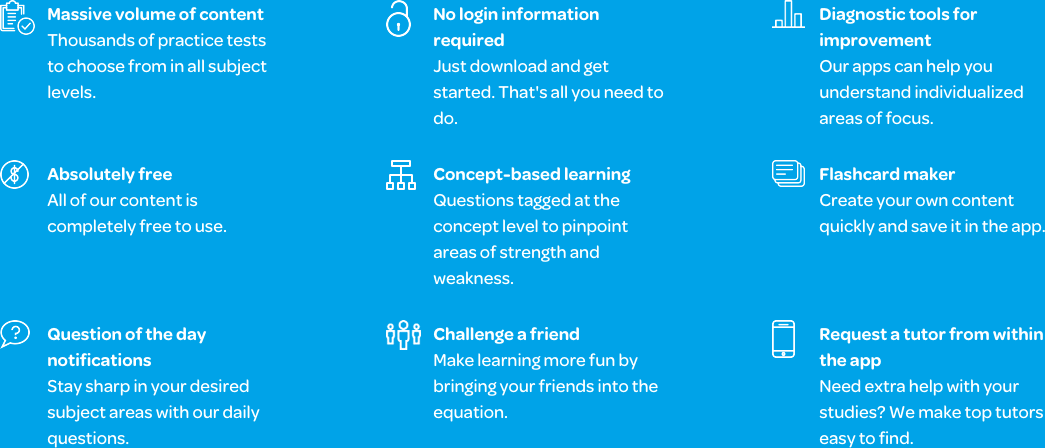# Varsity Tutors AP Calculus AB mobile app### The Varsity Tutors AP Calculus AB Mobile App

The Varsity Tutors AP Calculus AB app for Android, iPad, and iPhone is available on iTunes and the Google Play Store. It provides you with tools to help you prepare for the multiple-choice and free response sections of the exam. The app includes challenging full-length practice tests that can guide you through each concept and problem.

The app tests your knowledge of graphs, functions, lines, and derivatives and integrals. Other pertinent topics in AP Calculus AB include the limits and rules of differentiation, which will help you find the derivative of a function. Integration is another major topic, and you’ll learn techniques of varying complexities. Some applications include identifying the volumes of given solids. You are often tasked with finding the right method for making the calculation, which is something you are sure to encounter on the exam.

Section I of the test contains two parts. Part A has 28 questions that you’ll have 55 minutes to answer. Part A does not allow you to use a calculator. On Part B, 17 questions must be answered within 50 minutes. On this section, you may use a graphing calculator. Section II is free response, and you will have 90 minutes to work on its two parts. Part A has two problems that you will need to solve in 30 minutes, and you’ll be able to use a graphing calculator. Part B has four problems to be answered within one hour without the use of a calculator.

The exam is challenging, but fortunately the Varsity Tutors AP Calculus AB app is available to help shore up any uncertainties you might have about the material. You can access pre-made flashcards on your mobile device, or create unique flashcards with your own pictures, audio, and text.

The AP Calculus AB app for Android, iPhone, and iPad also lets you practice solving complex problems that will be presented on the free response portion of the test. You’ll be able to use a graphing calculator on these questions, but they may be tricky. It’s important to get as much practice in as possible, especially since you’ll have to answer four more without a calculator on the real test.

As you progress, the full-length practice tests will prove useful in studying. The Tests Taken feature will help you to track your results over time. In addition to testing your abilities, confidence, and endurance, you can also share the results with friends, tutors, and family members.

Use the AP Calculus AB app’s features to practice all the concepts you’ll need to know before the test. The app is designed to supplement what you learn in class. Though the content of the test is challenging, time management is just as important as answering the questions correctly. All of the multiple-choice questions are worth the same amount of points, so it’s possible to take an educated guess and still be on track. The more practice you get, the more prepared you will feel. This is just one of the many benefits provided by the Varsity Tutors AP Calculus AB app, which is available for Apple and Android devices on iTunes and the Google Play Store.If you are proficient in math, AP Calculus AB may be the high school course for you. This Advanced Placement track follows precalculus and may be your first introduction to calculus. It opens the door to many other AP math classes and tests. The full-year course includes content that many students don’t see until attending a college or university. You could receive college credit or have a placement advantage for successfully completing the course.

The general equivalent of a semester of college calculus, AP Calculus AB focuses mainly on differentiation and integration. It includes lots of graphical analysis; some components you’ll encounter are asymptotes and limits, derivatives, and integrals. By the time you start the course, you should have already mastered algebra, geometry, and trigonometry. The goals of the course are for you to understand how to work with functions, the meaning of a derivative related to rate of change and linear approximation, and the limit and change of a definite integral. Written and verbal communication, technology, and reasoning are essential skills that enable you to succeed in AP Calculus AB.

The AP Calculus AB Exam is about three hours and fifteen minutes. Its two parts each account for 50 percent of the final grade. The first section consists of 45 multiple-choice questions. You have one hour and forty-five minutes to work on Parts A and B. In Part A, there are 28 questions, and Part B has 17 questions. The main difference between the two is you cannot use a calculator for the first part, but a graphing calculator is permitted for the second part. Section 2 is free response and has six questions, which you have an hour and thirty minutes to complete. Part A of Section 2 has two problems, which you can use a graphing calculator to answer. The four problems of Section 2’s Part B must be answered on your own, without a calculator.

Generally, the AP Calculus AB curriculum starts with functions, graphs, and limits. You will need to master analyzing data on graphs before continuing, and will have to predict and explain the behavior of a function. The limits of functions are covered next. To calculate limits, you’ll need to apply the algebra learned in previous math courses, and graphs and tabulated data will be used to estimate the limits you are provided with. Other topics include asymptotic and unbounded behavior. Asymptotes on a graph indicate the infinite properties of limits and the rates of change of functions, accounting for exponential growth, logarithmic growth, and polynomial growth. Continuity is another important topic, in terms of limits and identifying the graphs for continuous functions.

You’ll also be introduced to the concept of the derivative and its relation to rate of change. The graphical, numerical, and analytical properties of derivatives will come into play. As you move forward, you will have to understand the derivative at a point, applying this to concepts such as the slope of a curve, for example. Calculations that will have to be made include approximating rate of change and calculating a tangent line to a curve. Working with a derivative of a function, more graphs will be used, and you’ll have to understand relationships between increasing and decreasing functions, denoted as the symbols f and f’. Concepts such as the Mean Value Theorem will be applied. You will also encounter equations with derivatives and translate verbal descriptions into such equations.

Other AP Calculus AB topics include second derivatives, which have traits such as concavity, and applications of derivatives. At this point, you will be called on to analyze curves, model rates of change, and find a derivative of an inverse function. Other aspects include absolute and local extrema. Geometric interpretation of differential equations is part of this stage of the course, along with computation of derivatives with functions such as exponential, power, trigonometric, inverse trigonometric, and logarithmic functions. Moving forward will also require understanding the chain rule and implicit differentiation.

Next, you will work with integrals. This section of the curriculum involves the properties of definite integrals and the applications of integrals in physics, biology, and economics. You will have to approximate Riemann sums, and use a definite integral to represent their limits. The Fundamental Theorem of Calculus comes next. With this, you’ll be able to evaluate definite integrals and represent antiderivatives. The theorem effectively links the concept of a function’s derivative with that of its integral. Its first part says the definite integration of a function, related to the antiderivative, can be reversed through differentiation. In the second part, the idea is that an infinite number of antiderivatives can be used to calculate a definite integral of a function. Sound hard? First understand the theorem simplifies the process of computing definite integrals, and is thus a key concept to consider as you move through AP Calculus AB.

The techniques and applications of antidifferentiation will be introduced next and, using Riemann sums, numerical approximations of definite integrals will have to be made. Techniques include working with antiderivatives following derivatives of functions, and by substitution of variables. Calculations will apply left, right, and midpoint evaluation points, plus trapezoidal sums to approximate the definite integrals of functions. Graphical, tabulated, and algebraic data are used to do this.

Yet another important AP Calculus AB topic is L’Hôpital’s rule, which provides further insight into derivatives, helping evaluate them with indeterminate forms. It also works with one-sided limits and involves the functions of f and g.

The principles of calculus are increasingly taking a role in students’ lives. More are taking AP Calculus exams; the number of test takers has increased to over 250,000 per year. The curriculum of AP Calculus AB is more advanced than what most high schoolers are used to, but if you meet the qualifications and prerequisites, it may be something to seriously consider. There are many complex topics to understand, but the benefits to the hard work can last through college and beyond. Taking on the challenge now may help set you apart in the future.